# Reminder and quotient

There are given the number C = 281, D = 201. Find the highest natural number S so that the C:S, D:S are with the remainder of 1,

Result

S =  40

#### Solution:Leave us a comment of example and its solution (i.e. if it is still somewhat unclear...):

Showing 0 comments:Be the first to comment!#### To solve this verbal math problem are needed these knowledge from mathematics:

Do you want to calculate greatest common divisor two or more numbers? Need help calculate sum, simplify or multiply fractions? Try our fraction calculator.

## Next similar examples:

1. Reminder and quotientThere are given numbers A = 135, B = 315. Find the smallest natural number R greater than 1 so that the proportions R:A, R:B are with the remainder 1.
2. GroupsIn the 6th class there are 60 girls and 72 boys. We want to divide them into groups so that the number of girls and boys is the same. How many groups can you create? How many girls will be in the group?
3. Sum of two primesChristian Goldbach, a mathematician, found out that every even number greater than 2 can be expressed as a sum of two prime numbers. Write or express 2018 as a sum of two prime numbers.
4. Primes 2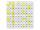Which prime numbers is number 2025 divisible?
5. MO C–I–1 2018An unknown number is divisible by just four numbers from the set {6, 15, 20, 21, 70}. Determine which ones.
6. TissuesThe store got three kinds of tissues - 132 children, 156 women and 204 men. Tissues each species were packed into boxes after the number of pieces the same for all three types (and greatest). Determine the number, if you know that every box has more than 6
7. Digit sumDetermine for how many integers greater than 900 and less than 1,001 has digit sum digit of the digit sum number 1.
8. Divisibility 2How many divisors has integer number 13?
9. The balls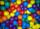You have 108 red and 180 green balls. You have to be grouped into the bags so that the ratio of red to green in each bag was the same. What smallest number of balls may be in one bag?
10. Six-digit primesFind all six-digit prime numbers that contain each one of digits 1,2,4,5,7 and 8 just once. How many are they?
11. Florist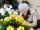Florist has 84 red and 48 white roses. How many same bouquets can he make from them if he must use all roses?
12. Hens and pigs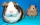Hens and pigs have 46 feet in total. At least how much can heads have?
13. One hundred stamps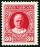A hundred letter stamps cost a hundred crowns. Its costs are four levels - twenty tenths , one crown, two-crown and five-crown. How many are each type of stamps? How many does the problem have solutions?
14. Product of two fractionsProduct of two fractions is 9 3/5 . If one of the fraction is 9 3/7. Find the other fraction.
15. PipeSteel pipe has a length 2.5 meters. About how many decimetres is 1/3 less than 4/8 of this steel pipe?
16. Pizza 5You have 2/4 of a pizza and you want to share it equally between 2 people how much pizza does each person get?
17. Fractions 4How many 2/3s are in 6?